# Quickstart¶

In this notebook, we go over the main functionalities of the library:

We will only show some minimal “get started” examples here. For more in depth information, you can refer to our user guide and example notebooks.

# Installing Darts¶

We recommend using some virtual environment. Then there are mainly two ways.

With pip:

pip install darts


With conda

conda install -c conda-forge -c pytorch u8darts-all


Consult the detailed install guide if you run into issues or want to install a different flavour (avoiding certain dependencies).

First, let’s import a few things:

:

%load_ext autoreload
%matplotlib inline

import pandas as pd
import numpy as np
import matplotlib.pyplot as plt

from darts import TimeSeries
from darts.datasets import AirPassengersDataset


# Building and manipulating TimeSeries¶

TimeSeries is the main data class in Darts. A TimeSeries represents a univariate or multivariate time series, with a proper time index. The time index can either be of type pandas.DatetimeIndex (containing datetimes), or of type pandas.RangeIndex (containing integers; useful for representing sequential data without specific timestamps). In some cases, TimeSeries can even represent probabilistic series, in order for instance to obtain confidence intervals. All models in Darts consume TimeSeries and produce TimeSeries.

## Read data and build a TimeSeries¶

TimeSeries can be built easily using a few factory methods:

• From an entire Pandas DataFrame, using TimeSeries.from_dataframe() (docs).

• From a time index and an array of corresponding values, using TimeSeries.from_times_and_values() (docs).

• From a NumPy array of values, using TimeSeries.from_values() (docs).

• From a Pandas Series, using TimeSeries.from_series() (docs).

• From an xarray.DataArray, using TimeSeries.from_xarray() (docs).

• From a CSV file, using TimeSeries.from_csv() (docs).

Below, we get a TimeSeries by directly loading the air passengers series from one of the datasets available in Darts:

:

series = AirPassengersDataset().load()
series.plot()## Some TimeSeries Operations¶

TimeSeries support different kinds of operations - here are a few examples.

splitting

We can also split at a fraction of the series, at a pandas Timestamp or at an integer index value.

:

series1, series2 = series.split_before(0.75)
series1.plot()
series2.plot()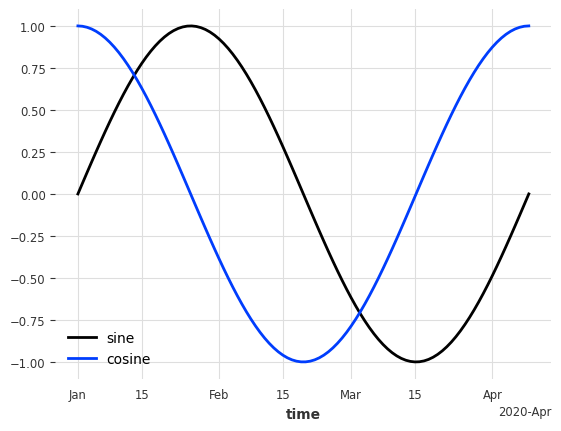slicing:

:

series1, series2 = series[:-36], series[-36:]
series1.plot()
series2.plot()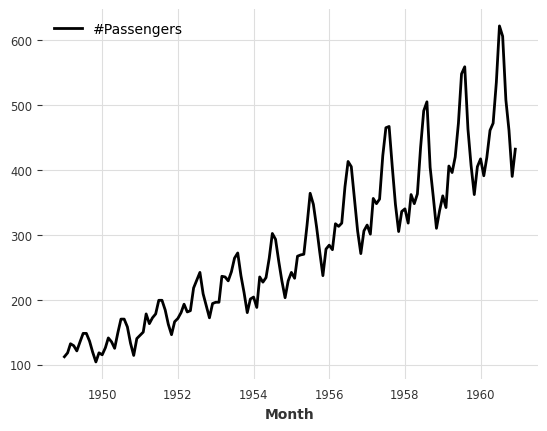arithmetic operations:

:

series_noise = TimeSeries.from_times_and_values(
series.time_index, np.random.randn(len(series))
)
(series / 2 + 20 * series_noise - 10).plot()stacking

Concatenating a new dimension to produce a new single multivariate series.

:

(series / 50).stack(series_noise).plot()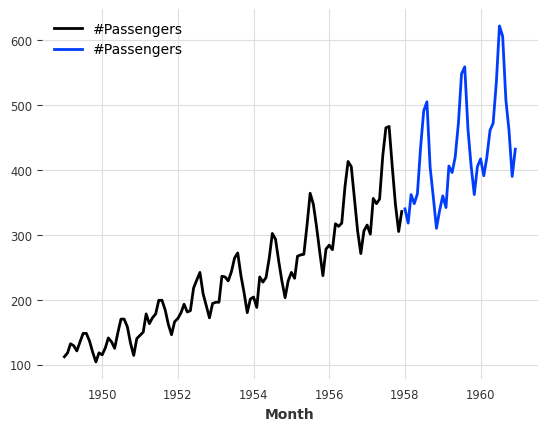mapping:

:

series.map(np.log).plot()mapping on both timestamps and values:

:

series.map(lambda ts, x: x / ts.days_in_month).plot()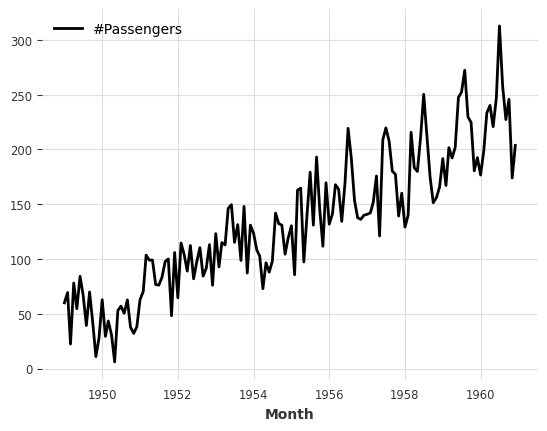Adding some datetime attribute as an extra dimension (yielding a multivariate series):

:

(series / 20).add_datetime_attribute("month").plot()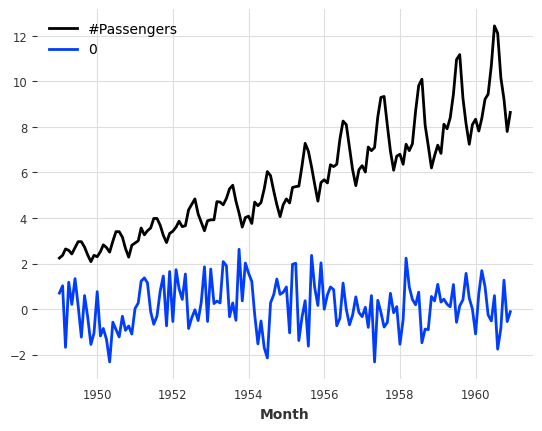:

(series / 200).add_holidays("US").plot()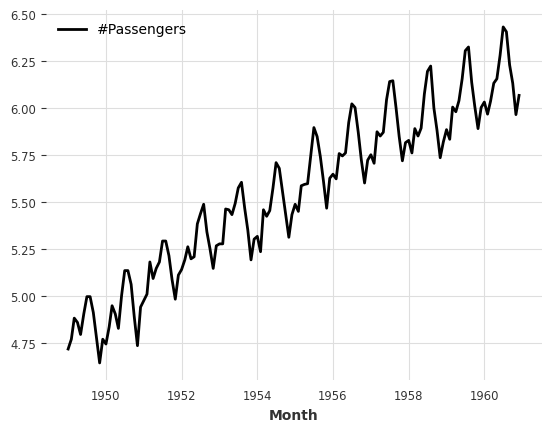differencing:

:

series.diff().plot()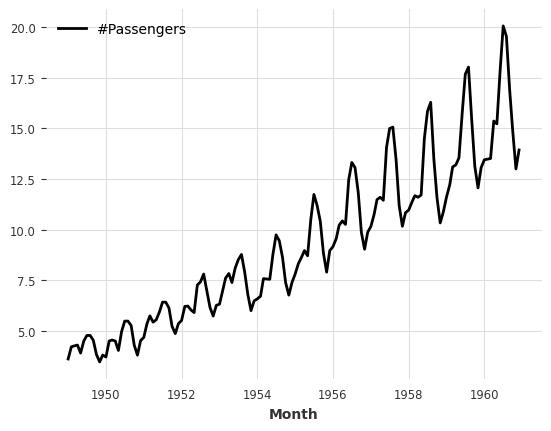Filling missing values (using a utils function).

Missing values are represented by np.nan.

:

from darts.utils.missing_values import fill_missing_values

values = np.arange(50, step=0.5)
values[10:30] = np.nan
values[60:95] = np.nan
series_ = TimeSeries.from_values(values)

(series_ - 10).plot(label="with missing values (shifted below)")
fill_missing_values(series_).plot(label="without missing values")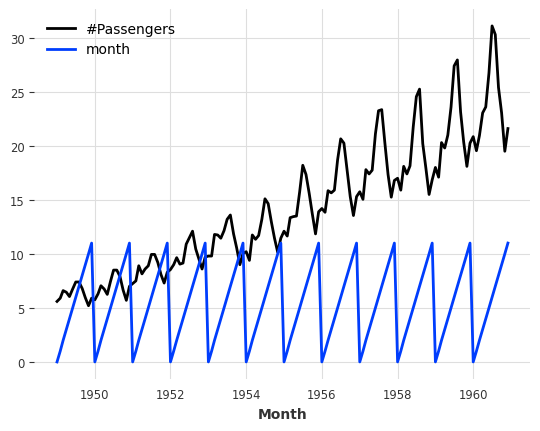## Creating a training and validation series¶

For what follows, we will split our TimeSeries into a training and a validation series. Note: in general, it is also a good practice to keep a test series aside and never touch it until the end of the process. Here, we just build a training and a validation series for simplicity.

The training series will be a TimeSeries containing values until January 1958 (excluded), and the validation series a TimeSeries containing the rest:

:

train, val = series.split_before(pd.Timestamp("19580101"))
train.plot(label="training")
val.plot(label="validation")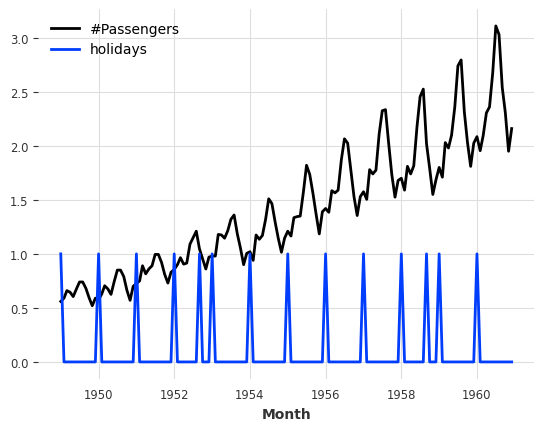# Training forecasting models and making predictions¶

## Playing with toy models¶

There is a collection of “naive” baseline models in Darts, which can be very useful to get an idea of the bare minimum accuracy that one could expect. For example, the NaiveSeasonal(K) model always “repeats” the value that occured K time steps ago.

In its most naive form, when K=1, this model simply always repeats the last value of the training series:

:

from darts.models import NaiveSeasonal

naive_model = NaiveSeasonal(K=1)
naive_model.fit(train)
naive_forecast = naive_model.predict(36)

series.plot(label="actual")
naive_forecast.plot(label="naive forecast (K=1)")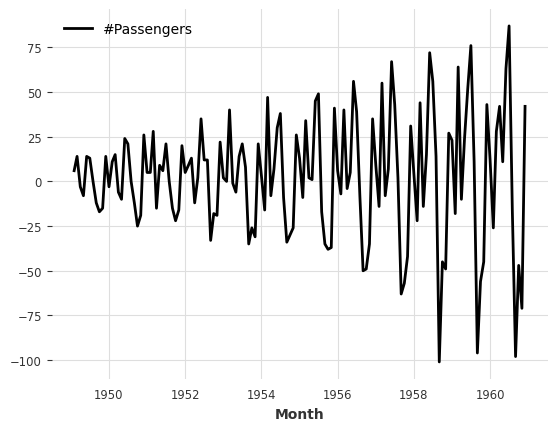It’s very easy to fit models and produce predictions on TimeSeries. All the models have a fit() and a predict() function. This is similar to Scikit-learn, except that it is specific to time series. The fit() function takes in argument the training time series on which to fit the model, and the predict() function takes in argument the number of time steps (after the end of the training series) over which to forecast.

### Inspect Seasonality¶

Our model above is perhaps a bit too naive. We can already improve by exploiting the seasonality in the data. It seems quite obvious that the data has a yearly seasonality, which we can confirm by looking at the auto-correlation function (ACF), and highlighting the lag m=12:

:

from darts.utils.statistics import plot_acf, check_seasonality

plot_acf(train, m=12, alpha=0.05)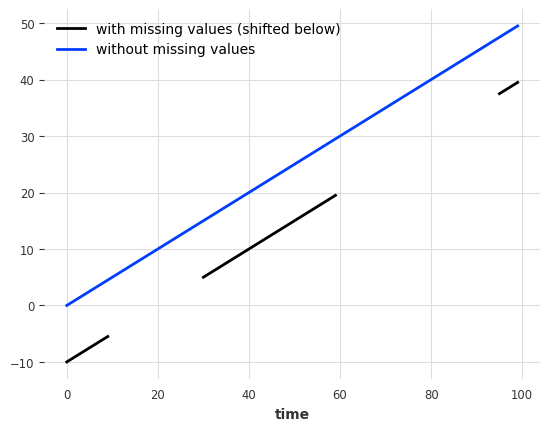The ACF presents a spike at x = 12, which suggests a yearly seasonality trend (highlighted in red). The blue zone determines the significance of the statistics for a confidence level of $$\alpha = 5\%$$. We can also run a statistical check of seasonality for each candidate period m:

:

for m in range(2, 25):
is_seasonal, period = check_seasonality(train, m=m, alpha=0.05)
if is_seasonal:
print("There is seasonality of order {}.".format(period))

There is seasonality of order 12.


### A less naive model¶

Let’s try the NaiveSeasonal model again with a seasonality of 12:

:

seasonal_model = NaiveSeasonal(K=12)
seasonal_model.fit(train)
seasonal_forecast = seasonal_model.predict(36)

series.plot(label="actual")
seasonal_forecast.plot(label="naive forecast (K=12)")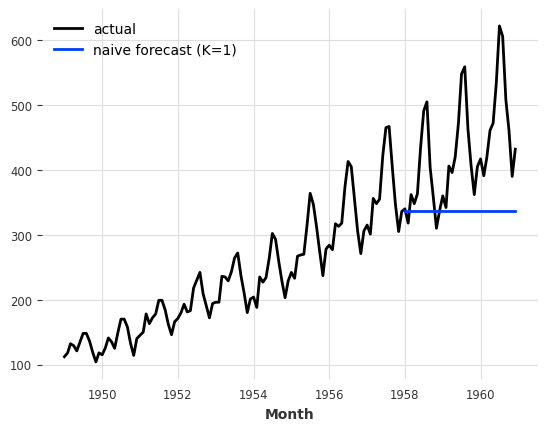This is better, but we are still missing the trend. Fortunately, there is also another naive baseline model capturing the trend, which is called NaiveDrift. This model simply produces linear predictions, with a slope that is determined by the first and last values of the training set:

:

from darts.models import NaiveDrift

drift_model = NaiveDrift()
drift_model.fit(train)
drift_forecast = drift_model.predict(36)

combined_forecast = drift_forecast + seasonal_forecast - train.last_value()

series.plot()
combined_forecast.plot(label="combined")
drift_forecast.plot(label="drift")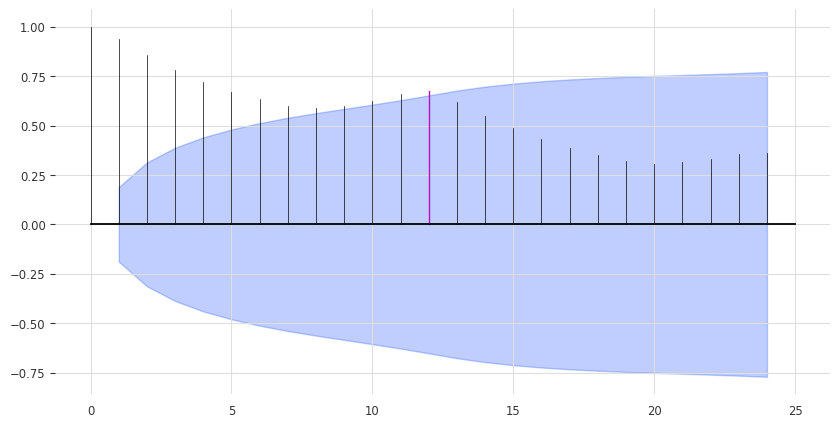What happened there? We simply fit a naive drift model, and add its forecast to the seasonal forecast we had previously. We also subtract the last value of the training set from the result, so that the resulting combined forecast starts off with the right offset.

## Computing error metrics¶

This looks already like a fairly decent forecast, and we did not use any non-naive model yet. In fact - any model should be able to beat this.

So what’s the error we will have to beat? We will use the Mean Absolute Percentage Error (MAPE) (note that in practice there are often good reasons not to use the MAPE - we use it here as it is quite convenient and scale independent). In Darts it is a simple function call:

:

from darts.metrics import mape

print(
"Mean absolute percentage error for the combined naive drift + seasonal: {:.2f}%.".format(
mape(series, combined_forecast)
)
)

Mean absolute percentage error for the combined naive drift + seasonal: 5.66%.


darts.metrics contains many more metrics to compare time series. The metrics will compare only common slices of series when the two series are not aligned, and parallelize computation over a large number of pairs of series - but let’s not get ahead of ourselves.

## Quickly try out several models¶

Darts is built to make it easy to train and validate several models in a unified way. Let’s train a few more and compute their respective MAPE on the validation set:

:

from darts.models import ExponentialSmoothing, TBATS, AutoARIMA, Theta

def eval_model(model):
model.fit(train)
forecast = model.predict(len(val))
print("model {} obtains MAPE: {:.2f}%".format(model, mape(val, forecast)))

eval_model(ExponentialSmoothing())
eval_model(TBATS())
eval_model(AutoARIMA())
eval_model(Theta())

model ExponentialSmoothing(trend=ModelMode.ADDITIVE, damped=False, seasonal=SeasonalityMode.ADDITIVE, seasonal_periods=12 obtains MAPE: 5.11%
model (T)BATS obtains MAPE: 5.87%
model Auto-ARIMA obtains MAPE: 11.65%
model Theta(2) obtains MAPE: 8.15%


Here, we did only built these models with their default parameters. We can probably do better if we fine-tune to our problem. Let’s try with the Theta method.

### Searching for hyper-parameters with the Theta method¶

The model Theta contains an implementation of Assimakopoulos and Nikolopoulos’ Theta method. This method has had some success, particularly in the M3-competition.

Though the value of the Theta parameter is often set to 0 in applications, our implementation supports a variable value for parameter tuning purposes. Let’s try to find a good value for Theta:

:

# Search for the best theta parameter, by trying 50 different values
thetas = 2 - np.linspace(-10, 10, 50)

best_mape = float("inf")
best_theta = 0

for theta in thetas:
model = Theta(theta)
model.fit(train)
pred_theta = model.predict(len(val))
res = mape(val, pred_theta)

if res < best_mape:
best_mape = res
best_theta = theta

:

best_theta_model = Theta(best_theta)
best_theta_model.fit(train)
pred_best_theta = best_theta_model.predict(len(val))

print(
"The MAPE is: {:.2f}, with theta = {}.".format(
mape(val, pred_best_theta), best_theta
)
)

The MAPE is: 4.40, with theta = -3.5102040816326543.

:

train.plot(label="train")
val.plot(label="true")
pred_best_theta.plot(label="prediction")We can observe that the model with best_theta is so far the best we have, in terms of MAPE.

# Backtesting: simulate historical forecasting¶

So at this point we have a model that performs well on our validation set, and that’s good. But, how can we know the performance we would have obtained if we had been using this model historically?

Backtesting simulates predictions that would have been obtained historically with a given model. It can take a while to produce, since the model is (by default) re-trained every time the simulated prediction time advances.

Such simulated forecasts are always defined with respect to a forecast horizon, which is the number of time steps that separate the prediction time from the forecast time. In the example below, we simulate forecasts done for 3 months in the future (compared to prediction time). The result of calling historical_forecasts() is (by default) a TimeSeries that contains those 3-months ahead forecasts:

:

historical_fcast_theta = best_theta_model.historical_forecasts(
series, start=0.6, forecast_horizon=3, verbose=True
)

series.plot(label="data")
print("MAPE = {:.2f}%".format(mape(historical_fcast_theta, series)))

MAPE = 7.70%So it seems that our best model on validation set is not doing so great anymore when we backtest it (did I hear overfitting :D)

To have a closer look at the errors, we can also use the backtest() method to obtain all the raw errors (say, MAPE errors) that would have been obtained by our model:

:

best_theta_model = Theta(best_theta)

raw_errors = best_theta_model.backtest(
series, start=0.6, forecast_horizon=3, metric=mape, reduction=None, verbose=True
)

from darts.utils.statistics import plot_hist

plot_hist(
raw_errors,
bins=np.arange(0, max(raw_errors), 1),
title="Individual backtest error scores (histogram)",
)Finally, using backtest() we can also get a simpler view of the average error over the historical forecasts:

:

average_error = best_theta_model.backtest(
series,
start=0.6,
forecast_horizon=3,
metric=mape,
reduction=np.mean,  # this is actually the default
verbose=True,
)

print("Average error (MAPE) over all historical forecasts: %.2f" % average_error)

Average error (MAPE) over all historical forecasts: 6.36


We could also for instance have specified the argument reduction=np.median to get the median MAPE instead.

Let’s look at the fitted value residuals of our current Theta model, i.e. the difference between the 1-step forecasts at every point in time obtained by fitting the model on all previous points, and the actual observed values:

:

from darts.utils.statistics import plot_residuals_analysis

plot_residuals_analysis(best_theta_model.residuals(series))We can see that the distribution is not centered at 0, which means that our Theta model is biased. We can also make out a large ACF value at lag equal to 12, which indicates that the residuals contain information that was not used by the model.

Could we maybe do better with a simple ExponentialSmoothing model?

:

model_es = ExponentialSmoothing()
historical_fcast_es = model_es.historical_forecasts(
series, start=0.6, forecast_horizon=3, verbose=True
)

series.plot(label="data")
historical_fcast_es.plot(label="backtest 3-months ahead forecast (Exp. Smoothing)")
print("MAPE = {:.2f}%".format(mape(historical_fcast_es, series)))

MAPE = 4.45%This much better! We get a mean absolute percentage error of about 4-5% when backtesting with a 3-months forecast horizon in this case.

:

plot_residuals_analysis(model_es.residuals(series))The residual analysis also reflects an improved performance in that we now have a distribution of the residuals centred at value 0, and the ACF values, although not insignificant, have lower magnitudes.

# Machine learning and global models¶

Darts has a rich support for machine learning and deep learning forecasting models; for instance:

• RegressionModel can wrap around any sklearn-compatible regression model to produce forecasts (it has its own section below).

• RNNModel is a flexible RNN implementation, which can be used like DeepAR.

• NBEATSModel implements the N-BEATS model.

• TFTModel implements the Temporal Fusion Transformer model.

• TCNModel implements temporal convolutional networks.

In addition to supporting the same basic fit()/predict() interface as the other models, these models are also global models, as they support being trained on multiple time series (sometimes referred to as meta learning).

This is a key point of using ML-based models for forecasting: more often than not, ML models (especially deep learning models) need to be trained on large amounts of data, which often means a large amount of separate yet related time series.

In Darts, the basic way to specify multiple TimeSeries is using a Sequence of TimeSeries (for instance, a simple list of TimeSeries).

## A toy example with two series¶

These models can be trained on thousands of series. Here, for the sake of illustration, we will load two distinct series - the air traffic passenger count and another series containing the number of pounds of milk produced per cow monthly. We also cast our series to np.float32 as that will slightly speedup the training:

:

from darts.datasets import AirPassengersDataset, MonthlyMilkDataset

# set aside last 36 months of each series as validation set:
train_air, val_air = series_air[:-36], series_air[-36:]
train_milk, val_milk = series_milk[:-36], series_milk[-36:]

train_air.plot()
val_air.plot()
train_milk.plot()
val_milk.plot()First, let’s scale these two series between 0 and 1, as that will benefit most ML models. We will use a Scaler for this:

:

from darts.dataprocessing.transformers import Scaler

scaler = Scaler()
train_air_scaled, train_milk_scaled = scaler.fit_transform([train_air, train_milk])

train_air_scaled.plot()
train_milk_scaled.plot()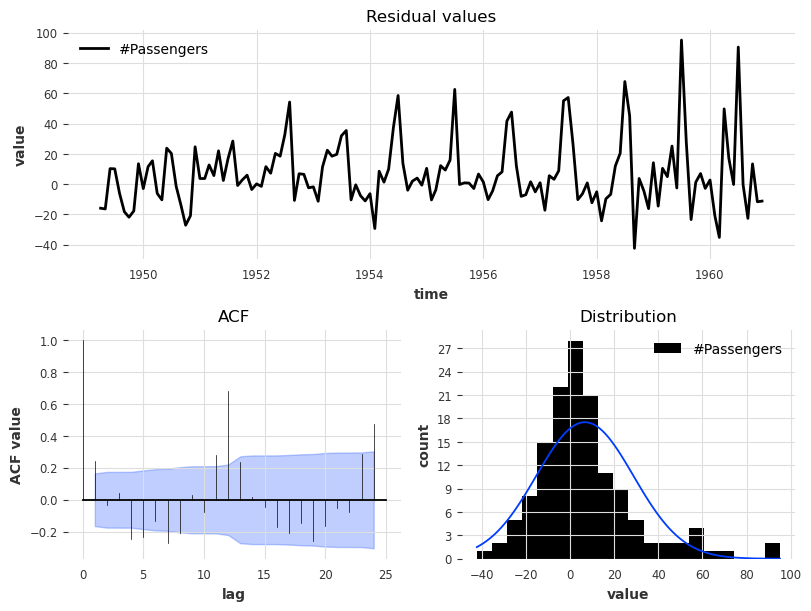Note how we can scale several series in one go. We can also parallelize this sort of operations over multiple processors by specifying n_jobs.

## Using deep learning: example with N-BEATS¶

Next, we will build an N-BEATS model. This model can be tuned with many hyper-parameters (such as number of stacks, layers, etc). Here, for simplicity, we will use it with default hyper-parameters. The only two hyper-parameters that we have to provide are:

• input_chunk_length: this is the “lookback window” of the model - i.e., how many time steps of history the neural network takes as input to produce its output in a forward pass.

• output_chunk_length: this is the “forward window” of the model - i.e., how many time steps of future values the neural network outputs in a forward pass.

The random_state parameter is just here to get reproducible results.

Most neural networks in Darts require these two parameters. Here, we will use multiples of the seasonality. We are now ready to fit our model on our two series (by giving a list containing the two series to fit()):

:

from darts.models import NBEATSModel

model = NBEATSModel(input_chunk_length=24, output_chunk_length=12, random_state=42)

model.fit([train_air_scaled, train_milk_scaled], epochs=50, verbose=True);

[2022-06-21 16:04:42,259] INFO | darts.models.forecasting.torch_forecasting_model | Train dataset contains 194 samples.
[2022-06-21 16:04:42,259] INFO | darts.models.forecasting.torch_forecasting_model | Train dataset contains 194 samples.
2022-06-21 16:04:42 darts.models.forecasting.torch_forecasting_model INFO: Train dataset contains 194 samples.
[2022-06-21 16:04:42,341] INFO | darts.models.forecasting.torch_forecasting_model | Time series values are 32-bits; casting model to float32.
[2022-06-21 16:04:42,341] INFO | darts.models.forecasting.torch_forecasting_model | Time series values are 32-bits; casting model to float32.
2022-06-21 16:04:42 darts.models.forecasting.torch_forecasting_model INFO: Time series values are 32-bits; casting model to float32.
2022-06-21 16:04:42 pytorch_lightning.utilities.rank_zero INFO: GPU available: False, used: False
2022-06-21 16:04:42 pytorch_lightning.utilities.rank_zero INFO: TPU available: False, using: 0 TPU cores
2022-06-21 16:04:42 pytorch_lightning.utilities.rank_zero INFO: IPU available: False, using: 0 IPUs
2022-06-21 16:04:42 pytorch_lightning.utilities.rank_zero INFO: HPU available: False, using: 0 HPUs
2022-06-21 16:04:42 pytorch_lightning.callbacks.model_summary INFO:
| Name          | Type             | Params
---------------------------------------------------
0 | criterion     | MSELoss          | 0
1 | train_metrics | MetricCollection | 0
2 | val_metrics   | MetricCollection | 0
3 | stacks        | ModuleList       | 6.2 M
---------------------------------------------------
6.2 M     Trainable params
1.4 K     Non-trainable params
6.2 M     Total params
24.787    Total estimated model params size (MB)


Let’s now get some forecasts 36 months ahead, for our two series. We can just use the series argument of the fit() function to tell the model which series to forecast. Importantly, the output_chunk_length does not directly constrain the forecast horizon n that can be used with predict(). Here, we trained the model with output_chunk_length=12 and produce forecasts for n=36 months ahead; this is simply done in an auto-regressive way behind the scenes (where the network recursively consumes its previous outputs).

:

pred_air = model.predict(series=train_air_scaled, n=36)
pred_milk = model.predict(series=train_milk_scaled, n=36)

# scale back:
pred_air, pred_milk = scaler.inverse_transform([pred_air, pred_milk])

plt.figure(figsize=(10, 6))
series_air.plot(label="actual (air)")
series_milk.plot(label="actual (milk)")
pred_air.plot(label="forecast (air)")
pred_milk.plot(label="forecast (milk)")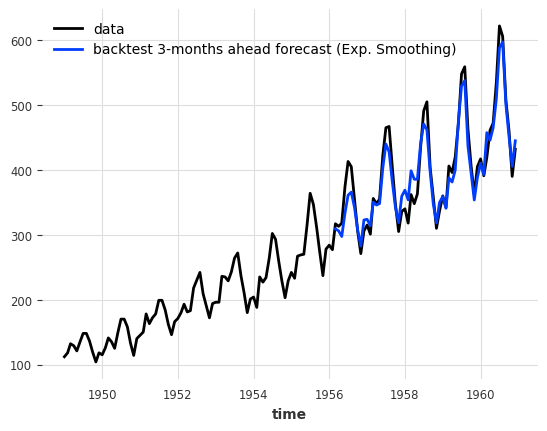Our forecasts are actually not so terrible, considering that we use one model with default hyper-parameters to capture both air passengers and milk production!

The model seems quite OK at capturing the yearly seasonality, but misses the trend for the air series. In the next section, we will try to solve this issue using external data (covariates).

# Covariates: using external data¶

In addition to the target series (the series we are interested to forecast), many models in Darts also accept covariates series in input. Covariates are series that we do not want to forecast, but which can provide helpful additional information to the models. Both the targets and covariates can be multivariate or univariate.

There are two kinds of covariate time series in Darts:

• past_covariates are series not necessarily known ahead of the forecast time. Those can for instance represent things that have to be measured and are not known upfront. Models do not use the future values of past_covariates when making forecasts.

• future_covariates are series which are known in advance, up to the forecast horizon. This can represent things such as calendar information, holidays, weather forecasts, etc. Models that accept future_covariates will look at the future values (up to the forecast horizon) when making forecasts.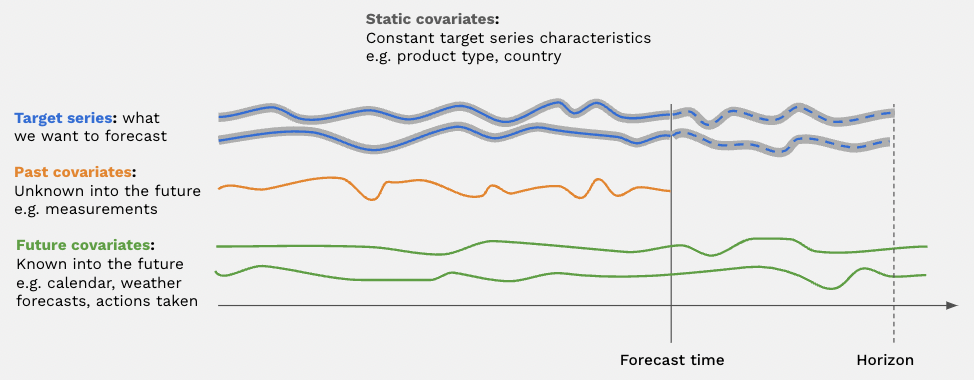Each covariate can potentially be multivariate. If you have several covariate series (such as month and year values), you should stack() or concatenate() them to obtain a multivariate series.

The covariates you provide can be longer than necessary. Darts will try to be smart and slice them in the right way for forecasting the target, based on the time indexes of the different series. You will receive an error if your covariates do not have a sufficient time span, though.

Let’s now build some external covariates containing both monthly and yearly values for our air and milk series. In the cell below, we use the darts.utils.timeseries_generation.datetime_attribute_timeseries() function to generate series containing the month and year values, and we concatenate() these series along the "component" axis in order to obtain one covariate series with two components (month and year), per target series. For simplicity, we directly scale the month and year values to have them between (roughly) 0 and 1:

:

from darts import concatenate
from darts.utils.timeseries_generation import datetime_attribute_timeseries as dt_attr

air_covs = concatenate(
[
dt_attr(series_air.time_index, "month", dtype=np.float32) / 12,
(dt_attr(series_air.time_index, "year", dtype=np.float32) - 1948) / 12,
],
axis="component",
)

milk_covs = concatenate(
[
dt_attr(series_milk.time_index, "month", dtype=np.float32) / 12,
(dt_attr(series_milk.time_index, "year", dtype=np.float32) - 1962) / 13,
],
axis="component",
)

air_covs.plot()
plt.title(
"one multivariate time series of 2 dimensions, containing covariates for the air series:"
);Not all models support all types of covariates. NBEATSModel supports only past_covariates. Therefore, even though our covariates represent calendar information and are known in advance, we will use them as past_covariates with N-BEATS. To train, all we have to do is give them as past_covariates to the fit() function, in the same order as the targets:

:

model = NBEATSModel(input_chunk_length=24, output_chunk_length=12, random_state=42)

model.fit(
[train_air_scaled, train_milk_scaled],
past_covariates=[air_covs, milk_covs],
epochs=50,
verbose=True,
);

[2022-06-21 16:05:10,026] INFO | darts.models.forecasting.torch_forecasting_model | Train dataset contains 194 samples.
[2022-06-21 16:05:10,026] INFO | darts.models.forecasting.torch_forecasting_model | Train dataset contains 194 samples.
2022-06-21 16:05:10 darts.models.forecasting.torch_forecasting_model INFO: Train dataset contains 194 samples.
[2022-06-21 16:05:10,085] INFO | darts.models.forecasting.torch_forecasting_model | Time series values are 32-bits; casting model to float32.
[2022-06-21 16:05:10,085] INFO | darts.models.forecasting.torch_forecasting_model | Time series values are 32-bits; casting model to float32.
2022-06-21 16:05:10 darts.models.forecasting.torch_forecasting_model INFO: Time series values are 32-bits; casting model to float32.
2022-06-21 16:05:10 pytorch_lightning.utilities.rank_zero INFO: GPU available: False, used: False
2022-06-21 16:05:10 pytorch_lightning.utilities.rank_zero INFO: TPU available: False, using: 0 TPU cores
2022-06-21 16:05:10 pytorch_lightning.utilities.rank_zero INFO: IPU available: False, using: 0 IPUs
2022-06-21 16:05:10 pytorch_lightning.utilities.rank_zero INFO: HPU available: False, using: 0 HPUs
2022-06-21 16:05:10 pytorch_lightning.callbacks.model_summary INFO:
| Name          | Type             | Params
---------------------------------------------------
0 | criterion     | MSELoss          | 0
1 | train_metrics | MetricCollection | 0
2 | val_metrics   | MetricCollection | 0
3 | stacks        | ModuleList       | 6.6 M
---------------------------------------------------
6.6 M     Trainable params
1.7 K     Non-trainable params
6.6 M     Total params
26.314    Total estimated model params size (MB)


Then to produce forecasts, we again have to provide our covariates as past_covariates to the predict() function. Even though the covariates time series also contains “future” values of the covariates up to the forecast horizon, the model will not consume those future values, because it uses them as past covariates (and not future covariates).

:

pred_air = model.predict(series=train_air_scaled, past_covariates=air_covs, n=36)
pred_milk = model.predict(series=train_milk_scaled, past_covariates=milk_covs, n=36)

# scale back:
pred_air, pred_milk = scaler.inverse_transform([pred_air, pred_milk])

plt.figure(figsize=(10, 6))
series_air.plot(label="actual (air)")
series_milk.plot(label="actual (milk)")
pred_air.plot(label="forecast (air)")
pred_milk.plot(label="forecast (milk)")It seems that now the model captures better the trend of the air series (which also perturbs a bit the forecasts of the milk series).

Using covariates related to the calendar or time axis (such as months and years as in our example above) is so frequent that deep learning models in Darts have a built-in functionality to use such covariates out of the box.

To easily integrate such covariates to your model, you can simply specify the add_encoders parameter at model creation. This parameter has to be a dictionary containing informations about what should be encoded as extra covariates. Here is an example of what such a dictionary could look like, for a model supporting both past and future covariates:

:

encoders = {
"cyclic": {"future": ["month"]},
"datetime_attribute": {"future": ["hour", "dayofweek"]},
"position": {"past": ["absolute"], "future": ["relative"]},
"custom": {"past": [lambda idx: (idx.year - 1950) / 50]},
"transformer": Scaler(),
}


In the above dictionary, the following things are specified:

• The month should be used as a future covariate, with a cyclic (sin/cos) encoding.

• The hour and day-of-the-week should be used as future covariates.

• The absolute position (time step in the series) should be used as past covariates.

• The relative position (w.r.t the forecasting time) should be used as future covariates.

• An additional custom function of the year should be used as past covariates.

• All the above covariates should be scaled using a Scaler, which will be fit upon calling the model fit() function and used afterwards to transform the covariates.

To replicate our example with month and year used as past covariates with N-BEATS, we can use some encoders as follows:

:

encoders = {"datetime_attribute": {"past": ["month", "year"]}, "transformer": Scaler()}


Now, the whole training of the N-BEATS model with these covariates looks as follows:

:

model = NBEATSModel(
input_chunk_length=24,
output_chunk_length=12,
random_state=42,
)

model.fit([train_air_scaled, train_milk_scaled], epochs=50, verbose=True);

[2022-06-21 16:05:39,967] INFO | darts.models.forecasting.torch_forecasting_model | Train dataset contains 194 samples.
[2022-06-21 16:05:39,967] INFO | darts.models.forecasting.torch_forecasting_model | Train dataset contains 194 samples.
2022-06-21 16:05:39 darts.models.forecasting.torch_forecasting_model INFO: Train dataset contains 194 samples.
[2022-06-21 16:05:40,031] INFO | darts.models.forecasting.torch_forecasting_model | Time series values are 32-bits; casting model to float32.
[2022-06-21 16:05:40,031] INFO | darts.models.forecasting.torch_forecasting_model | Time series values are 32-bits; casting model to float32.
2022-06-21 16:05:40 darts.models.forecasting.torch_forecasting_model INFO: Time series values are 32-bits; casting model to float32.
2022-06-21 16:05:40 pytorch_lightning.utilities.rank_zero INFO: GPU available: False, used: False
2022-06-21 16:05:40 pytorch_lightning.utilities.rank_zero INFO: TPU available: False, using: 0 TPU cores
2022-06-21 16:05:40 pytorch_lightning.utilities.rank_zero INFO: IPU available: False, using: 0 IPUs
2022-06-21 16:05:40 pytorch_lightning.utilities.rank_zero INFO: HPU available: False, using: 0 HPUs
2022-06-21 16:05:40 pytorch_lightning.callbacks.model_summary INFO:
| Name          | Type             | Params
---------------------------------------------------
0 | criterion     | MSELoss          | 0
1 | train_metrics | MetricCollection | 0
2 | val_metrics   | MetricCollection | 0
3 | stacks        | ModuleList       | 6.6 M
---------------------------------------------------
6.6 M     Trainable params
1.7 K     Non-trainable params
6.6 M     Total params
26.314    Total estimated model params size (MB)


And get some forecasts for the air passengers series:

:

pred_air = model.predict(series=train_air_scaled, n=36)

# scale back:
pred_air = scaler.inverse_transform(pred_air)

plt.figure(figsize=(10, 6))
series_air.plot(label="actual (air)")
pred_air.plot(label="forecast (air)")# Regression forecasting models¶

RegressionModel’s are forecasting models which wrap around sklearn-compatible regression models. The inner regression model is used to predict future values of the target series, as a function of certain lags of the target, past and future covariates. Behind the scenes, the time series are tabularized in order to build a training dataset in the right format.

By default, the RegressionModel will do a linear regression. It is very easy to use any desired sklearn-compatible regression model by specifying the model parameter, but for convenience Darts also provides a couple of ready-made models out of the box:

• RandomForest wraps around sklearn.ensemble.RandomForestRegressor.

• LightGBMModel wraps around lightbm.

• LinearRegressionModel wraps around sklearn.linear_model.LinearRegression (accepting the same kwargs).

For example, this is what fitting a Bayesian ridge regression to our toy two-series problem looks like:

:

from darts.models import RegressionModel
from sklearn.linear_model import BayesianRidge

model = RegressionModel(lags=72, lags_future_covariates=[-6, 0], model=BayesianRidge())

model.fit(
[train_air_scaled, train_milk_scaled], future_covariates=[air_covs, milk_covs]
);


Several things happened above:

• lags=72 is telling the RegressionModel to look at the past 72 lags of the target.

• In addition, lags_future_covariates=[-6, 0] means that the model will also look at lags of the future_covariates we provide. Here we enumerate the precise lags we want the models to take into account; the “-6th” and the “0th” lags. The “0th” lag means the “current” lag (i.e., at the time step being forecasted); obviously, knowning this lag requires knowing the data in advance (hence the fact we are using future_covariates). Similarly, -6 means we also look at the value of the covariates 6 months before the forecasted time step (which also requires to know the covariates in advance if we are forecasting at a horizon more than 6 steps ahead).

• model=BayesianRidge() provides the actual inner regression model.

Now let’s get some forecasts:

:

pred_air, pred_milk = model.predict(
series=[train_air_scaled, train_milk_scaled],
future_covariates=[air_covs, milk_covs],
n=36,
)

# scale back:
pred_air, pred_milk = scaler.inverse_transform([pred_air, pred_milk])

plt.figure(figsize=(10, 6))
series_air.plot(label="actual (air)")
series_milk.plot(label="actual (milk)")
pred_air.plot(label="forecast (air)")
pred_milk.plot(label="forecast (milk)")Note how we obtained the forecasts for the two time series at once above. Similarly, we can also get some metrics over sequences of series:

:

mape([series_air, series_milk], [pred_air, pred_milk])

:

[3.41736301779747, 5.282935127615929]


or the average metric over “all” series:

:

mape([series_air, series_milk], [pred_air, pred_milk], inter_reduction=np.mean)

:

4.350149072706699


By the way: similarly to transformers such as Scaler, computing metrics can be parallelized over N processors when executed over many series pairs by specifying n_jobs=N.

It seems that this model performs well on the Air traffic series, how does it do when we backtest it on this one series?

:

bayes_ridge_model = RegressionModel(
lags=72, lags_future_covariates=, model=BayesianRidge()
)

backtest = bayes_ridge_model.historical_forecasts(
series_air, future_covariates=air_covs, start=0.6, forecast_horizon=3, verbose=True
)

print("MAPE = %.2f" % (mape(backtest, series_air)))
series_air.plot()
backtest.plot()

MAPE = 3.66Our best model so far!

# Probabilistic forecasts¶

Some models can produce probabilistic forecasts. This is the case for all deep learning models (such as RNNModel, NBEATSModel, etc …), as well as for ARIMA and ExponentialSmoothing. The full list is available on the Darts README page.

For ARIMA and ExponentialSmoothing, one can simply specify a num_samples parameter to the predict() function. The returned TimeSeries will then be composed of num_samples Monte Carlo samples describing the distribution of the time series’ values. The advantage of relying on Monte Carlo samples (in contrast to, say, explicit confidence intervals) is that they can be used to describe any parametric or non-parametric joint distribution over components, and compute arbitrary quantiles.

:

model_es = ExponentialSmoothing()
model_es.fit(train)
probabilistic_forecast = model_es.predict(len(val), num_samples=500)

series.plot(label="actual")
probabilistic_forecast.plot(label="probabilistic forecast")
plt.legend()
plt.show()## With neural networks¶

With neural networks, one has to give a Likelihood object to the model. The likelihoods specify which distribution the model will try to fit, along with potential prior values for the distributions’ parameters. The full list of available likelihoods is available in the docs.

Using likelihoods is easy. For instance, here is what training an NBEATSModel to fit a Laplace likelihood looks like:

:

from darts.models import TCNModel
from darts.utils.likelihood_models import LaplaceLikelihood

model = TCNModel(
input_chunk_length=24,
output_chunk_length=12,
random_state=42,
likelihood=LaplaceLikelihood(),
)

model.fit(train_air_scaled, epochs=400, verbose=True);

[2022-06-21 16:06:11,849] INFO | darts.models.forecasting.torch_forecasting_model | Train dataset contains 73 samples.
[2022-06-21 16:06:11,849] INFO | darts.models.forecasting.torch_forecasting_model | Train dataset contains 73 samples.
2022-06-21 16:06:11 darts.models.forecasting.torch_forecasting_model INFO: Train dataset contains 73 samples.
[2022-06-21 16:06:11,853] INFO | darts.models.forecasting.tcn_model | Number of layers chosen: 3
[2022-06-21 16:06:11,853] INFO | darts.models.forecasting.tcn_model | Number of layers chosen: 3
2022-06-21 16:06:11 darts.models.forecasting.tcn_model INFO: Number of layers chosen: 3
[2022-06-21 16:06:11,856] INFO | darts.models.forecasting.torch_forecasting_model | Time series values are 32-bits; casting model to float32.
[2022-06-21 16:06:11,856] INFO | darts.models.forecasting.torch_forecasting_model | Time series values are 32-bits; casting model to float32.
2022-06-21 16:06:11 darts.models.forecasting.torch_forecasting_model INFO: Time series values are 32-bits; casting model to float32.
2022-06-21 16:06:11 pytorch_lightning.utilities.rank_zero INFO: GPU available: False, used: False
2022-06-21 16:06:11 pytorch_lightning.utilities.rank_zero INFO: TPU available: False, using: 0 TPU cores
2022-06-21 16:06:11 pytorch_lightning.utilities.rank_zero INFO: IPU available: False, using: 0 IPUs
2022-06-21 16:06:11 pytorch_lightning.utilities.rank_zero INFO: HPU available: False, using: 0 HPUs
2022-06-21 16:06:11 pytorch_lightning.callbacks.model_summary INFO:
| Name          | Type              | Params
----------------------------------------------------
0 | criterion     | MSELoss           | 0
1 | train_metrics | MetricCollection  | 0
2 | val_metrics   | MetricCollection  | 0
3 | dropout       | MonteCarloDropout | 0
4 | res_blocks    | ModuleList        | 166
----------------------------------------------------
166       Trainable params
0         Non-trainable params
166       Total params
0.001     Total estimated model params size (MB)


Then to get probabilistic forecasts, we again only need to specify some num_samples >> 1:

:

pred = model.predict(n=36, num_samples=500)

# scale back:
pred = scaler.inverse_transform(pred)

series_air.plot()
pred.plot()Furthermore, we could also for instance specify that we have some prior belief that the scale of the distribution is about $$0.1$$ (in the transformed domain), while still capturing some time dependency of the distribution, by specifying prior_b=.1.

Behind the scenes this will regularize the training loss with a Kullback-Leibler divergence term.

:

model = TCNModel(
input_chunk_length=24,
output_chunk_length=12,
random_state=42,
likelihood=LaplaceLikelihood(prior_b=0.1),
)

model.fit(train_air_scaled, epochs=400, verbose=True);

[2022-06-21 16:06:26,627] INFO | darts.models.forecasting.torch_forecasting_model | Train dataset contains 73 samples.
[2022-06-21 16:06:26,627] INFO | darts.models.forecasting.torch_forecasting_model | Train dataset contains 73 samples.
2022-06-21 16:06:26 darts.models.forecasting.torch_forecasting_model INFO: Train dataset contains 73 samples.
[2022-06-21 16:06:26,632] INFO | darts.models.forecasting.tcn_model | Number of layers chosen: 3
[2022-06-21 16:06:26,632] INFO | darts.models.forecasting.tcn_model | Number of layers chosen: 3
2022-06-21 16:06:26 darts.models.forecasting.tcn_model INFO: Number of layers chosen: 3
[2022-06-21 16:06:26,636] INFO | darts.models.forecasting.torch_forecasting_model | Time series values are 32-bits; casting model to float32.
[2022-06-21 16:06:26,636] INFO | darts.models.forecasting.torch_forecasting_model | Time series values are 32-bits; casting model to float32.
2022-06-21 16:06:26 darts.models.forecasting.torch_forecasting_model INFO: Time series values are 32-bits; casting model to float32.
2022-06-21 16:06:26 pytorch_lightning.utilities.rank_zero INFO: GPU available: False, used: False
2022-06-21 16:06:26 pytorch_lightning.utilities.rank_zero INFO: TPU available: False, using: 0 TPU cores
2022-06-21 16:06:26 pytorch_lightning.utilities.rank_zero INFO: IPU available: False, using: 0 IPUs
2022-06-21 16:06:26 pytorch_lightning.utilities.rank_zero INFO: HPU available: False, using: 0 HPUs
2022-06-21 16:06:26 pytorch_lightning.callbacks.model_summary INFO:
| Name          | Type              | Params
----------------------------------------------------
0 | criterion     | MSELoss           | 0
1 | train_metrics | MetricCollection  | 0
2 | val_metrics   | MetricCollection  | 0
3 | dropout       | MonteCarloDropout | 0
4 | res_blocks    | ModuleList        | 166
----------------------------------------------------
166       Trainable params
0         Non-trainable params
166       Total params
0.001     Total estimated model params size (MB)

:

pred = model.predict(n=36, num_samples=500)

# scale back:
pred = scaler.inverse_transform(pred)

series_air.plot()
pred.plot()By default TimeSeries.plot() shows the median as well as the 5th and 95th percentiles (of the marginal distributions, if the TimeSeries is multivariate). It is possible to control this:

:

pred.plot(low_quantile=0.01, high_quantile=0.99, label="1-99th percentiles")
pred.plot(low_quantile=0.2, high_quantile=0.8, label="20-80th percentiles")### Types of distributions¶

The likelihood has to be compatible with the domain of your time series’ values. For instance PoissonLikelihood can be used on discrete positive values, ExponentialLikelihood can be used on real positive values, and BetaLikelihood on real values in $$(0,1)$$.

It is also possible to use QuantileRegression to apply a quantile loss and fit some desired quantiles directly.

### Evaluating Probabilistic Forecasts¶

How can we evaluate the quality of probabilistic forecasts? By default, most metrics functions (such as mape()) will keep working but look only at the median forecast. It is also possible to use the $$\rho$$-risk metric (or quantile loss), which quantifies the error for each predicted quantiles:

:

from darts.metrics import rho_risk

print("MAPE of median forecast: %.2f" % mape(series_air, pred))
for rho in [0.05, 0.1, 0.5, 0.9, 0.95]:
rr = rho_risk(series_air, pred, rho=rho)
print("rho-risk at quantile %.2f: %.2f" % (rho, rr))

MAPE of median forecast: 11.75
rho-risk at quantile 0.05: 0.14
rho-risk at quantile 0.10: 0.15
rho-risk at quantile 0.50: 0.11
rho-risk at quantile 0.90: 0.03
rho-risk at quantile 0.95: 0.02


### Using Quantile Loss¶

Could we do better by fitting these quantiles directly? We can just use a QuantileRegression likelihood:

:

from darts.utils.likelihood_models import QuantileRegression

model = TCNModel(
input_chunk_length=24,
output_chunk_length=12,
random_state=42,
likelihood=QuantileRegression([0.05, 0.1, 0.5, 0.9, 0.95]),
)

model.fit(train_air_scaled, epochs=400, verbose=True);

[2022-06-21 16:06:42,853] INFO | darts.models.forecasting.torch_forecasting_model | Train dataset contains 73 samples.
[2022-06-21 16:06:42,853] INFO | darts.models.forecasting.torch_forecasting_model | Train dataset contains 73 samples.
2022-06-21 16:06:42 darts.models.forecasting.torch_forecasting_model INFO: Train dataset contains 73 samples.
[2022-06-21 16:06:42,858] INFO | darts.models.forecasting.tcn_model | Number of layers chosen: 3
[2022-06-21 16:06:42,858] INFO | darts.models.forecasting.tcn_model | Number of layers chosen: 3
2022-06-21 16:06:42 darts.models.forecasting.tcn_model INFO: Number of layers chosen: 3
[2022-06-21 16:06:42,862] INFO | darts.models.forecasting.torch_forecasting_model | Time series values are 32-bits; casting model to float32.
[2022-06-21 16:06:42,862] INFO | darts.models.forecasting.torch_forecasting_model | Time series values are 32-bits; casting model to float32.
2022-06-21 16:06:42 darts.models.forecasting.torch_forecasting_model INFO: Time series values are 32-bits; casting model to float32.
2022-06-21 16:06:42 pytorch_lightning.utilities.rank_zero INFO: GPU available: False, used: False
2022-06-21 16:06:42 pytorch_lightning.utilities.rank_zero INFO: TPU available: False, using: 0 TPU cores
2022-06-21 16:06:42 pytorch_lightning.utilities.rank_zero INFO: IPU available: False, using: 0 IPUs
2022-06-21 16:06:42 pytorch_lightning.utilities.rank_zero INFO: HPU available: False, using: 0 HPUs
2022-06-21 16:06:42 pytorch_lightning.callbacks.model_summary INFO:
| Name          | Type              | Params
----------------------------------------------------
0 | criterion     | MSELoss           | 0
1 | train_metrics | MetricCollection  | 0
2 | val_metrics   | MetricCollection  | 0
3 | dropout       | MonteCarloDropout | 0
4 | res_blocks    | ModuleList        | 208
----------------------------------------------------
208       Trainable params
0         Non-trainable params
208       Total params
0.001     Total estimated model params size (MB)

:

pred = model.predict(n=36, num_samples=500)

# scale back:
pred = scaler.inverse_transform(pred)

series_air.plot()
pred.plot()

print("MAPE of median forecast: %.2f" % mape(series_air, pred))
for rho in [0.05, 0.1, 0.5, 0.9, 0.95]:
rr = rho_risk(series_air, pred, rho=rho)
print("rho-risk at quantile %.2f: %.2f" % (rho, rr))

MAPE of median forecast: 4.97
rho-risk at quantile 0.05: 0.00
rho-risk at quantile 0.10: 0.00
rho-risk at quantile 0.50: 0.03
rho-risk at quantile 0.90: 0.01
rho-risk at quantile 0.95: 0.01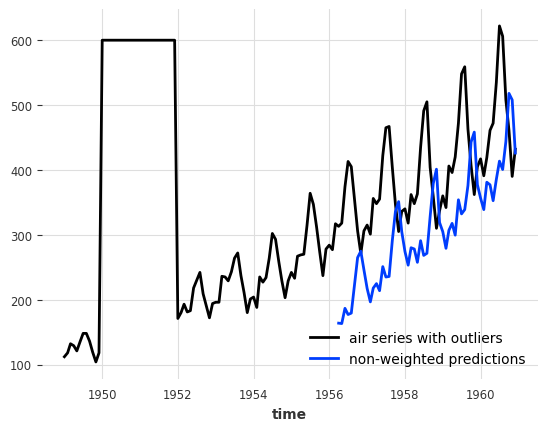# Ensembling models¶

Ensembling is about combining the forecasts produced by several models, in order to obtain a final - and hopefully better forecast.

For instance, in our example of a less naive model above, we manually combined a naive seasonal model with a naive drift model. Here, we will show how models forecasts can be automatically combined, naively using a NaiveEnsembleModel, or learned using RegressionEnsembleModel.

## Naive Ensembling¶

Naive ensembling just takes the average of the forecasts of several models. Darts provides a NaiveEnsembleModel, which allows to do this while still manipulating only one forecasting model (which, for instance, allows for easier backtesting):

:

from darts.models import NaiveEnsembleModel

models = [NaiveDrift(), NaiveSeasonal(12)]

ensemble_model = NaiveEnsembleModel(models=models)

backtest = ensemble_model.historical_forecasts(
series_air, start=0.6, forecast_horizon=3, verbose=True
)

print("MAPE = %.2f" % (mape(backtest, series_air)))
series_air.plot()
backtest.plot()

MAPE = 11.88## Learned Ensembling¶

As expected in this case, the naive ensemble doesn’t give great results (although in some cases it could!)

We can sometimes do better if we see the ensembling as a supervised regression problem: given a set of forecasts (features), find a model that combines them in order to minimise errors on the target. This is what the RegressionEnsembleModel does. It accepts three parameters:

• forecasting_models is a list of forecasting models whose predictions we want to ensemble.

• regression_train_n_points is the number of time steps to use for fitting the “ensemble regression” model (i.e., the inner model that combines the forecasts).

• regression_model is, optionally, a sklearn-compatible regression model or a Darts RegressionModel to be used for the ensemble regression. If not specified, a linear regression is used. Using a sklearn model is easy out-of-the-box, but using a RegressionModel allows to potentially take arbitrary lags of the individual forecasts as inputs of the regression model.

Once these elements are in place, a RegressionEnsembleModel can be used like a regular forecasting model:

:

from darts.models import RegressionEnsembleModel

models = [NaiveDrift(), NaiveSeasonal(12)]

ensemble_model = RegressionEnsembleModel(
forecasting_models=models, regression_train_n_points=12
)

backtest = ensemble_model.historical_forecasts(
series_air, start=0.6, forecast_horizon=3, verbose=True
)

print("MAPE = %.2f" % (mape(backtest, series_air)))
series_air.plot()
backtest.plot()

MAPE = 4.85We can also inspect the coefficients used to weigh the two inner models in the linear combination:

:

ensemble_model.regression_model.model.coef_

:

array([0.09948264, 1.0118142 ])


# Filtering models¶

In addition to forecasting models, which are able to predict future values of series, Darts also contains a couple of helpful filtering models, which can model “in sample” series’ values distributions.

## Fitting a Kalman Filter¶

KalmanFilter implements a Kalman Filter. The implementation relies on nfoursid, so it is for instance possible to provide a nfoursid.kalman.Kalman object containing a transition matrix, process noise covariance, observation noise covariance etc.

It is also possible to do system identification by calling fit() to “train” the Kalman Filter using the N4SID system identification algorithm:

:

from darts.models import KalmanFilter

kf = KalmanFilter(dim_x=3)
kf.fit(train_air_scaled)
filtered_series = kf.filter(train_air_scaled, num_samples=100)

train_air_scaled.plot()
filtered_series.plot()## Inferring missing values with Gaussian Processes¶

Darts also contains a GaussianProcessFilter which can be used for probabilistic modeling of series:

:

from darts.models import GaussianProcessFilter
from sklearn.gaussian_process.kernels import RBF

# create a series with holes:
values = train_air_scaled.values()
values[20:22] = np.nan
values[28:32] = np.nan
values[55:59] = np.nan
values[72:80] = np.nan
series_holes = TimeSeries.from_times_and_values(train_air_scaled.time_index, values)
series_holes.plot()

kernel = RBF()

gpf = GaussianProcessFilter(kernel=kernel, alpha=0.1, normalize_y=True)
filtered_series = gpf.filter(series_holes, num_samples=100)

filtered_series.plot()# A Word of Caution¶

So is N-BEATS, exponential smoothing, or a Bayesian ridge regression trained on milk production the best approach for predicting the future number of airline passengers? Well, at this point it’s actually hard to say exactly which one is best. Our time series is small, and our validation set is even smaller. In such cases, it’s very easy to overfit the whole forecasting exercise to such a small validation set. That’s especially true if the number of available models and their degrees of freedom is high (such as for deep learning models), or if we played with many models on a single test set (as done in this notebook).

As data scientists, it is our responsibility to understand the extent to which our models can be trusted. So always take results with a grain of salt, especially on small datasets, and apply the scientific method before making any kind of forecast :) Happy modeling!